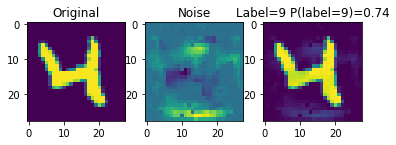This notebook demonstrates how easy it is to create adversarial examples. Let's start by training some models to classify the digits in the MNIST data set. We'll work with one fully-connected neural network (MLP) and one convolutional network to show the generality of our approach.

In :
import torch
import torch.nn as nn
import torch.nn.functional as F
import torch.optim as optim

from torchvision import datasets, transforms
import matplotlib.pyplot as plt

class FCNet(nn.Module):
def __init__(self):
super(FCNet, self).__init__()
self.layer1 = nn.Linear(28 * 28, 50)
self.layer2 = nn.Linear(50, 20)
self.layer3 = nn.Linear(20, 10)
def forward(self, img):
flattened = img.view(-1, 28 * 28)
activation1 = F.relu(self.layer1(flattened))
activation2 = F.relu(self.layer2(activation1))
output = self.layer3(activation2)
return output

class ConvNet(nn.Module):
def __init__(self):
super(ConvNet, self).__init__()
self.conv1 = nn.Conv2d(1, 5, 5, padding=2)
self.pool = nn.MaxPool2d(2, 2)
self.conv2 = nn.Conv2d(5, 10, 5, padding=2)
self.fc1 = nn.Linear(10 * 7 * 7, 32)
self.fc2 = nn.Linear(32, 10)

def forward(self, x):
x = self.pool(F.relu(self.conv1(x)))
x = self.pool(F.relu(self.conv2(x)))
x = x.view(-1, 10 * 7 * 7)
x = F.relu(self.fc1(x))
x = self.fc2(x)
x = x.squeeze(1)
return x

In :
def train(model, data, batch_size=64, lr=0.001, num_iters=1000, print_every=100):
optimizer = optim.Adam(model.parameters(), lr=lr)
criterion = nn.CrossEntropyLoss()

total_loss = 0
n = 0

while True:
for imgs, labels in iter(train_loader):
out = model(imgs)
loss = criterion(out, labels)
loss.backward()
optimizer.step()
total_loss += loss.item()
n += 1

if n % print_every == 0:
print("Iter %d. Avg.Loss: %f" % (n, total_loss/print_every))
total_loss = 0
if n > num_iters:
return

In :
fc_model = FCNet()
train(fc_model, mnist_images, num_iters=1000)

Iter 100. Avg.Loss: 1.411140
Iter 200. Avg.Loss: 0.528199
Iter 300. Avg.Loss: 0.444489
Iter 400. Avg.Loss: 0.342946
Iter 500. Avg.Loss: 0.350003
Iter 600. Avg.Loss: 0.325551
Iter 700. Avg.Loss: 0.322285
Iter 800. Avg.Loss: 0.322599
Iter 900. Avg.Loss: 0.268067
Iter 1000. Avg.Loss: 0.220982

In :
cnn_model = FCNet()
train(cnn_model, mnist_images, num_iters=1000)

Iter 100. Avg.Loss: 1.345714
Iter 200. Avg.Loss: 0.570480
Iter 300. Avg.Loss: 0.477422
Iter 400. Avg.Loss: 0.370881
Iter 500. Avg.Loss: 0.365108
Iter 600. Avg.Loss: 0.321058
Iter 700. Avg.Loss: 0.315379
Iter 800. Avg.Loss: 0.322854
Iter 900. Avg.Loss: 0.263115
Iter 1000. Avg.Loss: 0.219685


## Targetted Adversarial Attack¶

The purpose of an adversarial attack is to perturb an input (usually an image $x$) so that a neural network $f$ misclassifies the perturbed image $x + \epsilon$. In a targeted attack, we want the network $f$ to misclassify the perturbed image into a class of our choosing.

Let's begin with this image. We will perturb the image so our model thinks that the image is of the digit 3, when in fact it is of the digit 5.

In :
image = mnist_images
target_label = 3
model = fc_model

plt.imshow(image)

Out:
<matplotlib.image.AxesImage at 0x122b8a4e0>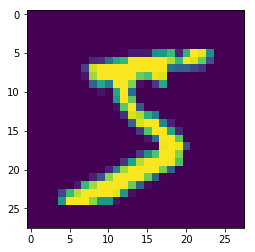Our approach is as follows:

• We will create a random noise $\epsilon$ that is the same size as the image.
• We will use an optimizer to tune the values of $\epsilon$ to make the neural network misclassify $x + \epsilon$ to our target class

The second step might sound a little mysterious, but is actually very similar to tuning the weights of a neural network!

First, let's create some noise values. In order for PyTorch to be able to tune these values using an optimizer, we need to set requires_grad=True:

In :
noise = torch.randn(1, 28, 28) * 0.01


Now, we will tune the noise:

In :
optimizer = optim.Adam([noise], lr=0.01, weight_decay=1)
criterion = nn.CrossEntropyLoss()

for i in range(1000):
adv_image = torch.clamp(image + noise, 0, 1)
loss = criterion(out, torch.Tensor([target_label]).long())
loss.backward()
optimizer.step()


To keep the pixel values in noise small, we use a fairly large weight_decay. We use the CrossEntropyLoss, but maximize the neural network prediction of our target_label.

Notice also that the adv_image is clamped so that the pixel values are kept in the range [0, 1].

Now, let's see the resulting image:

In :
adv_image = torch.clamp(image + noise, 0, 1)

plt.subplot(1, 3, 1)
plt.title("Original")
plt.imshow(image)

plt.subplot(1, 3, 2)
plt.title("Noise")
plt.imshow(noise.detach().numpy())

plt.subplot(1, 3, 3)

Out:
<matplotlib.image.AxesImage at 0x122bbbcc0>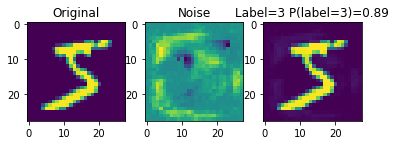The image on the right still looks like a "5" to a human. However, the neural network misclassifies the image.

The same steps can be used to create an adversarial attack for other images, and for other model architectures.

In :
def create_adversarial_example(model, image, target_label):
noise = torch.randn(1, 28, 28)

optimizer = optim.Adam([noise], lr=0.01, weight_decay=1)
criterion = nn.CrossEntropyLoss()

for i in range(1000):
adv_image = torch.clamp(image + noise, 0, 1)
loss = criterion(out, torch.Tensor([target_label]).long())
loss.backward()
optimizer.step()

adv_image = torch.clamp(image + noise, 0, 1)

plt.subplot(1, 3, 1)
plt.title("Original")
plt.imshow(image)

plt.subplot(1, 3, 2)
plt.title("Noise")
plt.imshow(noise.detach().numpy())

plt.subplot(1, 3, 3)

In :
create_adversarial_example(fc_model, mnist_images, 3)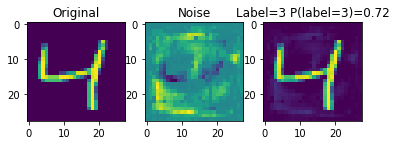In :
create_adversarial_example(cnn_model, mnist_images, 3)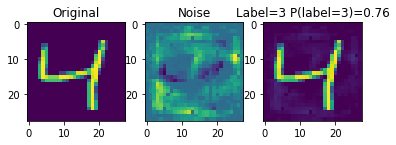In :
create_adversarial_example(fc_model, mnist_images, 9)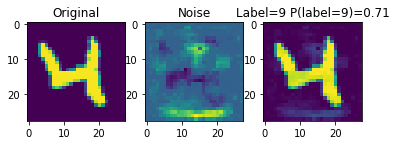In :
create_adversarial_example(cnn_model, mnist_images, 9)